## RD Sharma Class 9 Solutions Chapter 1 Number Systems Ex 1.3

These Solutions are part of RD Sharma Class 9 Solutions. Here we have given RD Sharma Class 9 Solutions Chapter 1 Number Systems Ex 1.3

Question 1.
Express each of the following decimals in the form $$\frac { p }{ q }$$:
(i) 0.39
(ii) 0.750
(iii) 2.15
(iv) 7.010
(v) 9.90
(vi) 1.0001
Solution:Question 2.
Express each of the following decimals in the form $$\frac { p }{ q }$$: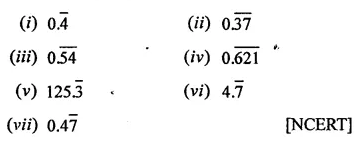Solution: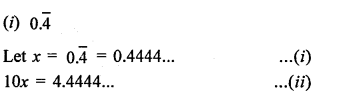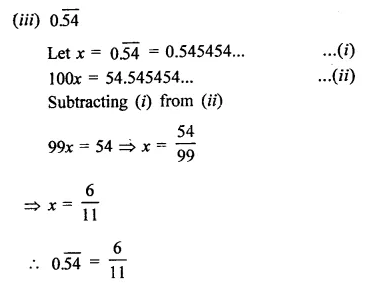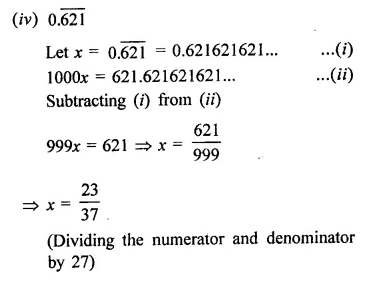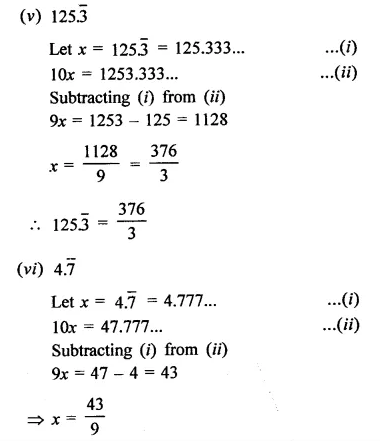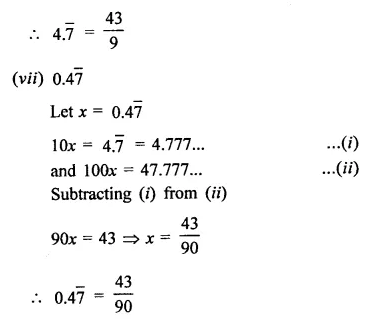Hope given RD Sharma Class 9 Solutions Chapter 1 Number Systems Ex 1.3 are helpful to complete your math homework.

If you have any doubts, please comment below. Learn Insta try to provide online math tutoring for you.